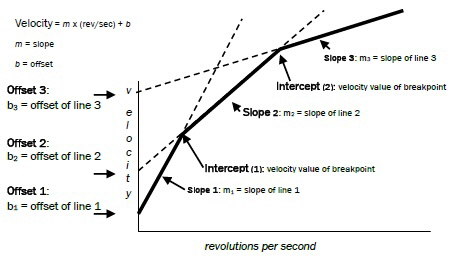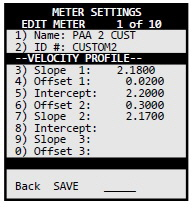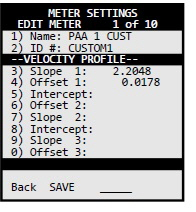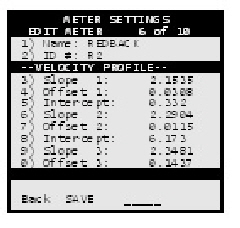<title> <META NAME="description" CONTENT="AquaCalc LLC / JBS Instruments specializes in stream flow measurement devices like the AquaCalc that are used in the field of hydrology."> <META NAME="keywords" CONTENT="jbs instruments aquacalc aquacount stream flow measurement water discharge current meter hydrology usgs"> </title>

# Using Non-Standard Current Meters including OTT type and Redback current meters

The AquaCalc has the current meter rating equations for many standard United States Geological Survey (USGS) meters built in when shipped to the user. The AquaCalc can also accept custom current meter equations for non-standard USGS and European type horizontal axis meters. Most contact type current meters can be used with the AquaCalc if the rating equation is known from calibration tests.

In addition to the built-in current meter rating curves for the Price AA and Pygmy meters, the AquaCalc can store rating curves for user defined “non-standard” current meters including OTT type meters and Hydrological Services Redback Price AA type current meter.

As used by the USGS, meter rating curves are either a single or pair of equations that define the relationship between the number of revolutions per second (counts divided by time) of the current meter cups and the measured velocity. These equations are use to create the meter rating tables used in manual measurements.

Non-standard current meter ratings can be defined in several segments, one of which is used for lower velocities, the other segments for progressively higher velocities. Each segment is represented by an equation. A crossover or “breakpoint” velocity value is also specified that indicates the velocity at which the next equation is used.

Each equation takes the form:

y = m * R + b

where R is the Revolutions per second

or

Velocity = m * (revolutions/second) + b

Where m is the slope of the line and b is the value where the line intercepts the velocity axis (which in the AquaCalc is identified as the “Offset”).

Velocity = Slope * (revolutions/second) + Offset

The AquaCalc can handle non-standard meter rating curves with up to three line segments representing three velocity ranges. The “Intercept” value in the AquaCalc represents the crossover or breakpoint velocity value at which second (or third) segment is used.

Figure : Current Meter Definition in the AquaCalcSo for a sample Price Type AA Standard No. 1 two segment equation example (This rating is no longer used and has been replaced with new Price Type AA Standard No. 2 below and is included as an example only):

EQUATIONS: V=2.18R + .020(2.200) 2.17R + .030

Note that the value in parenthesis (2.200) represents the velocity above which the second segment is used. The above equation would be entered into the second AquaCalc Meter Settings screen as follows:A single segment rating curve equation (such as the new Price Type AA Standard No. 2) would be entered in the second Meter Setup screen as:

EQUATION: V = 2.2048R + 0.0178## Custom Current Meter Rating for a Hydrological Services Redback Price AA style current meter

Until JBS implements the Hydrological Services Redback current meter as one of the standard ratings in the AquaCalc, existing AquaCalc Pro Plus (and Pro) users can enter the following values into any available “Non-Standard” custom meter in the Meters screen. Once entered, the custom meter is stored and available any time. (Note: In the AquaCalc meter setup screen, the velocity crossover is labeled as “Intercept”):

Hydrological Services has provided the following equations for the current meter rating for a Redback current meter:

n <= 0.14      V = 2.1535 n +0.0308 ft/s

0.14 < n < 2.69        V = 2.2904 n +0.0115 ft/s

n >= 2.69       V = 2.3481 n -0.1437 ft/s

Multipart USGS current meter ratings use a crossover velocity instead of the n = revolutions per second crossover point, but these are easily converted. From the table attached to your rating, the 0.14 rev/sec is equal to 0.332 ft/sec, and the 2.69 rev/sec is equal to 6.173 ft/sec.

The USGS traditionally uses a specific format for multipart ratings,  V= Slope1 * R + Offset1 (VC) Slope2 * R + Offset2, Where R is revolutions per second, and VC is the velocity at the crossover point.

Translating the Redback “Imperial” rating into the USGS format yields: V

V=2.1535R + 0.0308 (0.332) 2.2904R + 0.0115 (6.173) 2.3481R - 0.1437

And this is entered into the AquaCalc Pro Plus As

• Name: REDBACK
• ID #: 0-00E (Or as needed)
• Type: NonStd
•
• Slope 1:     2.1535
• Offset 1:    0.0308
• Intercept 1: 0.332
• Slope 2:     2.2904
• Offset 2:    0.0115
• Intercept 2: 6.173
• Slope 3:     2.3481
• Offset 3:  - 0.1437Step-by-step procedure to add a non-standard current meter

1. From the main menu, select number 4 “Meter Setup”
2. Use the right arrow navigation key to select a new meter to specify.
3. Press number 1 to name this meter
4. Press number 2 to name the meter ID number
5. Press number 3 to select the type of meter “NonStd”
6. Press number 4 to change to metric if required.
7. Press number 5 to change revolutions/pulses
8. Using the ‘2 of 2’ Soft-Key, go to page 2
9. Utilizing keypad number 3 through 0, enter meter constants
10. Press Save

Using Custom Meters in the AquaCalc Pro Plus

AquaCalc LLC. · telephone +1.916.372.0534 · e-mail: sales@aquacalc.com